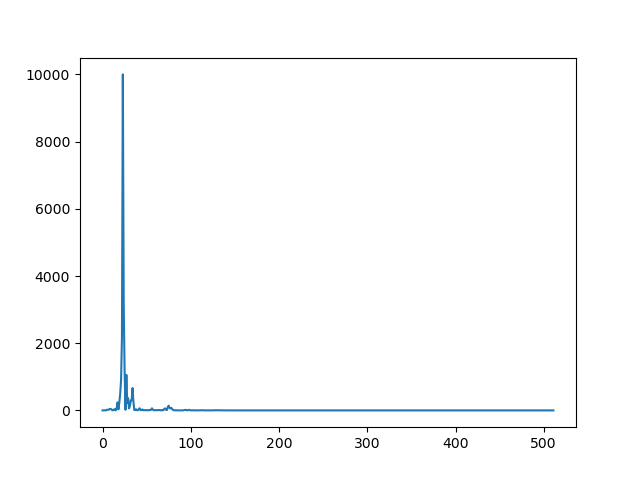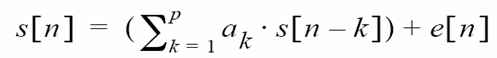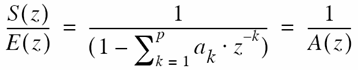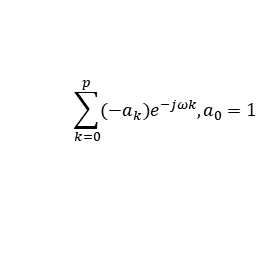www.b2bchain.cn

# Python语音信号特征-感知线性预测系数PLP

PLP基本流程：

1.Perceptual linear predictive (PLP) analysis of speech-H.Hermansk,1938-1952,1990
2.噪声条件下的语音特征PLP参数的提取-太原理工大学学报
3.https://blog.csdn.net/xmdxcsj/article/details/78512568

## Python代码

1.用到的安装包

``import numpy as np import wave import matplotlib.pyplot as plt import librosa``

2.读取.wav信号，进行预加重、分帧、加窗

``f = wave.open(r"04.wav","rb")#将.wav放在pycharm工程目录下,rb为只读 params = f.getparams() nchannels, sampwidth, framerate, nframes =params[:4] print(params[:4])#返回声道数、量化位数、采样频率、采样点数 str_data = f.readframes(nframes)#readframes返回的是字符串类型的数据 signal =np.fromstring(str_data,dtype=np.int16)#将字符串转换为int类型的数组，我的sampwidth为2，所以转换为int16 signal_len=len(signal) signal=signal/(max(abs(signal)))#归一化 ------预加重并画时域波形---------- signal_add=np.append(signal,signal[1:]-0.97*signal[:-1])    time=np.arange(0,nframes)/1.0*framerate #画时间轴 plt.figure(figsize=(20,10)) plt.subplot(2,1,1) plt.plot(time[0:len(signal)],signal)#可能出现时间轴和信号长度不相等的情况，我这里选取了与singala长度相等的time plt.xlabel('time') plt.subplot(2,1,2) plt.plot(time[0:len(signal_add)],signal_add) plt.xlabel('time') plt.show() ----分帧------ wlen=1024#每帧信号的帧数 inc=256#帧移 N=512#每帧信号帧数的一半 nf = int(np.ceil((1.0 * signal_len - wlen + inc) / inc))#计算帧数nf pad_len=int((nf-1)*inc+wlen) zeros=np.zeros(pad_len-signal_len)#不够的点用0填补 pad_signal=np.concatenate((signal,zeros)) indices=np.tile(np.arange(0,wlen)，(nf,1))+np.tile(np.arange(0,nf*inc,inc),(wlen,1)).T indices=np.array(indices,dtype=np.int32) frames=pad_signal[indices]#最终得到帧数*帧长形式的信号数据 win=np.hamming(wlen)#先调用窗函数 x=frames[10:] #我选取其中某一帧的数据提取PLP系数，即为frames的某一行 y=win*x#得到加窗后的信号``3.进行FFT，选取15个中心频点并进行滤波，得到15维的频带能量向量

``-----先定义了两个公式，bark_change为线性频率坐标转换为Bark坐标，equal_loudness为等响度曲线-------- def bark_change(x): return 6*np.log10(x/(1200*np.pi)+((x/(1200*np.pi))**2+1)**0.5) def equal_loudness(x):    return ((x**2+56.8e6)*x**4)/((x**2+6.3e6)**2*(x**2+3.8e8)) a=np.fft.fft(y)#做fft，默认为信号的长度即1024点 b=np.square(abs(a[0:N])) #求fft变换结果的模的平方，只取a的一半 df=framerate/N  #频率分辨率 i=np.arange(N)#只取大于0部分的频率 freq_hz=i*df#得到实际频率坐标 plt.plot(freq_hz,b)#得到该帧信号的功率谱 plt.show() freq_w=2*np.pi*np.array(freq_hz)#转换为角频率 freq_bark=bark_change(freq_w)#再转换为bark频率 point_hz= [250, 350, 450, 570, 700, 840,1000, 1170, 1370, 1600, 1850, 2150,2500,2900,3400] #选取的临界频带数量一般要大于10，覆盖常用频率范围，这里我选取了15个中心频点 point_w=2*np.pi*np.array(point_hz)#转换为角频率 point_bark =bark_change(point_w)#转换为bark频率 bank=np.zeros((15,N))#构造15行512列的矩阵，每一行为一个滤波器向量 filter_data=np.zeros(15)#构造15维频带能量向量 -------构造滤波器组，这一段注意缩进--------- for j in range(15):       for k in range(N):         omg= freq_bark[k]- point_bark[j]               if -1.3<omg<-0.5:           bank[j,k]=10**(2.5*(omg+0.5))               elif -0.5<omg<0.5:                      bank[j,k]=1             elif 0.5<omg<2.5:                      bank[j,k] = 10**(-1.0*(omg-0.5))               else:                      bank[j,k]=0       filter_data[j] = np.sum(b * bank[j]) #滤波后将该段信号相加，最终得到15维的频带能量         plt.plot(freq_hz,bank[j])    plt.xlim(0,20000)       plt.xlabel('hz') plt.show()#画滤波器组``4.等响曲线滤波，立方根压缩，IFFT

``equal_data=equal_loudness(point_w)*filter_data cubic_data=equal_data**0.33 ---做30点的ifft，得到30维PLP向量------------ plp_data=np.fft.ifft(cubic_data,30) print(plp_data)``

5.PLP谱包络和传统LPC谱包络对比

``---用librosa直接求传统LPC系数，需要0.7以上版本--- plp=librosa.lpc(abs(plp_data), 15)#要求输入参数为浮点型，经过ifft得到的plp_data有复数，因此要取abs h1=1.0/np.fft.fft(plp,1024) spec_envelope_plp=10*np.log10(abs(h1[0:512])) lpc=librosa.lpc(y,15) h2=1.0/np.fft.fft(lpc,1024) spec_envelope_lpc =10*np.log10(abs(h2[0:512])) plt.plot(spec_envelope_plp,'b',spec_envelope_lpc,'r') plt.show()``Z变换后，发现与源滤波模型中的声道传输函数相同，因此求出LP系数后，可以根据傅里叶变换求声道传输函数H=1./fft(A,N),A 为LP系数向量，N为fft点数，再由10log10(abs(H))求谱包络。• wave.Error: unknown format:3
.wav 文件的格式问题，需要转换成wave.open()中支持的的格式，因此需要格式的转变。需要在命令提示符窗口安装sox，然后输入sox原文件.wav -b 16 -e signed-integer 转变后的文件.wav
参考：https://blog.csdn.net/qq_31390999/article/details/95062506
• No module named ‘numba.decorators
由于我的librosa-0.7.2 与 numba-0.50.0版本不匹配，应该重新下载numba-0.48.0，于是我按照文章https://blog.csdn.net/July_Wander/article/details/106857289在命令提示符窗口pip uninstall numba又pip install numba-0.48.0，还是不行，在pycharm中File–>Settings->Project–>Project Interpreter中，我发现librosa的最新版本是0.8.0，于是我直接更新librosa，点击右侧▲标识即可。或者点击librosa这一行，勾选右下角specify version，再选择左下角install package，等待install successfully。再运行发现问题已经解决。

• 微信咨询
• 回顶